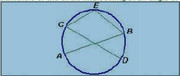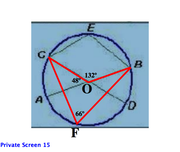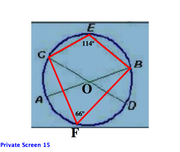GMAT Question of the Day - Daily to your Mailbox; hard ones only

 It is currently 15 Dec 2019, 08:19### GMAT Club Daily Prep

#### Thank you for using the timer - this advanced tool can estimate your performance and suggest more practice questions. We have subscribed you to Daily Prep Questions via email.

Customized
for You

we will pick new questions that match your level based on your Timer History

Track

every week, we’ll send you an estimated GMAT score based on your performance

Practice
Pays

we will pick new questions that match your level based on your Timer History

#### Not interested in getting valuable practice questions and articles delivered to your email? No problem, unsubscribe here.# In the figure AB and CD are two diameters of circle. Interse

Author Message
TAGS:

### Hide Tags

ManagerJoined: 03 Jan 2015
Posts: 72
Re: In the figure AB and CD are two diameters of circle. Interse  [#permalink]

### Show Tags

Could anyone please be so kind to explain this question with use of diagrams? I have a very hard time understanding the answer to this question. Thank you very much
DirectorP
Joined: 29 Jun 2017
Posts: 931
Re: In the figure AB and CD are two diameters of circle. Interse  [#permalink]

### Show Tags

we have to connect C to center O and prove that the angle at the center is double the angle CEB.

this is hard.
InternB
Joined: 14 Aug 2018
Posts: 20
Location: United States (WA)
GMAT 1: 670 Q43 V40GMAT 2: 750 Q47 V47GPA: 3.3
WE: Education (Education)
Re: In the figure AB and CD are two diameters of circle. Interse  [#permalink]

### Show Tags

Quick elimination tip here

An triangle formed by the diameter of a circle and any other point on that circle is a right triangle. The diameter is the hypotenuse and the right angle is formed by the two legs extending to the point. So, in this case, we can create a right triangle by drawing a line from, say, E to D. This shows that angle CED is greater than 90, so eliminate C, D and E.
_________________
Jayson Beatty
Indigo Prep
http://www.indigoprep.com
GMATH TeacherP
Status: GMATH founder
Joined: 12 Oct 2010
Posts: 935
Re: In the figure AB and CD are two diameters of circle. Interse  [#permalink]

### Show Tags

PathFinder007 wrote:
Attachment:
Circle_Angle.JPG
In the figure AB and CD are two diameters of circle. Intersecting at angle 48 degree. E is any point on Arc CB. find angle CEB

A. 114
B. 100
C. 80
D. 96
E. 40

All angles are measured in degrees.

$$? = x = \angle CEB$$01. Triangle AOC is isosceles with base AC, hence the 66-degrees angle is justified.

02. Angle BAC is inscribed in the circle, hence the 132-degrees red arc (CEB) is justified.

03. Angle x (our FOCUS) is inscribed in the circle and corresponds to the blue arc CADB, hence:

$$? = {{360 - 132} \over 2} = 114\,\,\,\,\,\,\,\, \Rightarrow \,\,\,\,\,\left( A \right)$$

This solution follows the notations and rationale taught in the GMATH method.

Regards,
Fabio.
_________________
Fabio Skilnik :: GMATH method creator (Math for the GMAT)
Our high-level "quant" preparation starts here: https://gmath.net
GMAT Club LegendV
Joined: 12 Sep 2015
Posts: 4157
Re: In the figure AB and CD are two diameters of circle. Interse  [#permalink]

### Show Tags

2
Top Contributor
PathFinder007 wrote:
Attachment:
Circle_Angle.JPG
In the figure AB and CD are two diameters of circle. Intersecting at angle 48 degree. E is any point on Arc CB. find angle CEB

A. 114
B. 100
C. 80
D. 96
E. 40

Let's use some useful circle properties

First let's add a blue line, to divide ∠CEB into 2 anglesCD is the DIAMETER of the circle
Since ∠CED is an inscribed angle containing (aka "holding") the diameter, we can conclude that ∠CED = 90°Now recognize that the intersection of AB and CD creates two equal (vertically opposite) angles, we can conclude the angle opposite the 48° is also 48°Now recognize that we have two angles containing (aka "holding") the arc BD
Property: If a CENTRAL angle and an INSCRIBED angle contain the same arc, then the CENTRAL angle is TWICE the INSCRIBED angle
This means that ∠DEB = 24°At this point, we have:So, ∠CEB = 90° + 24° = 114°

RELATED VIDEO FROM OUR COURSE

_________________
ManagerB
Joined: 07 Apr 2018
Posts: 100
Location: United States
Concentration: General Management, Marketing
GMAT 1: 600 Q45 V28GPA: 3.8
Re: In the figure AB and CD are two diameters of circle. Interse  [#permalink]

### Show Tags

Angle CBE + Angle ODB = 180 degree as sum of opposite angles of a quadrilateral is 180.
So, CBE =180-66=114
InternB
Joined: 18 Jul 2018
Posts: 18
Re: In the figure AB and CD are two diameters of circle. Interse  [#permalink]

### Show Tags

karishma,

I have a doubt..so from what I understand is that the angle around O should be 360 degree, so shouldnt angle COB be 132 degree? angle COA and angle AOD adds up to 180 degree. angle BOD is 48 degree, making it all add up to 228 degree. Then should angle COB not be 360-228=132 degree?Where am I going wrong, pls help

2nd doubt- is angle COA =48 degree? if not, why? (i assumed angle BOD and COA to be vertically opposite)
DirectorG
Joined: 04 Aug 2010
Posts: 505
Schools: Dartmouth College
Re: In the figure AB and CD are two diameters of circle. Interse  [#permalink]

### Show Tags

1
1
Quote:In the figure AB and CD are two diameters of circle. Intersecting at angle 48 degree. E is any point on Arc CB. find angle CEB

A. 114
B. 100
C. 80
D. 96
E. 40

An INSCRIBED ANGLE is formed by two chords.
A CENTRAL ANGLE is formed by two radii.
When an inscribed angle and a central angle intercept the same two points on a circle, the inscribed angle is 1/2 the central angle.Here, inscribed angle CFB and central angle COB both intercept the circle at points C and B.
Since central angle COB = 132º, inscribed angle CFB = (1/2)(132) = 66º.

Rule:
When a quadrilateral is inscribed in a circle, opposite angles sum to 180º.Since opposite angles inside inscribed quadrilateral CEBF must sum to 180º, angle CEB = 180-66 = 114.

_________________
GMAT and GRE Tutor
New York, NY

Available for tutoring in NYC and long-distance.
ManagerB
Joined: 02 Jan 2017
Posts: 58
Location: India
Schools: Oxford "21
Re: In the figure AB and CD are two diameters of circle. Interse  [#permalink]

### Show Tags

Shree9975 wrote:
Hi,

Can any1 explain me how angle COB=180 degrees. Why is the sum 180+48?

Angle COB is not 180.

Note that the angle around O will be 360 degrees. There is angle COB, the smaller angle (the angle that arc CEB subtends at the center) and angle COB, the larger angle (shown by red arrow around center O in the second diagram). The major arc CADB subtends larger angle COB at the center and inscribed angle CEB at the circle. The central angle will be twice the inscribed angle.

The larger angle COB = angle COA + angle AOD + angle DOB = 180 + 48 = 228 = Central angle

Inscribed angle = 228/2 = 114

Can uou please tell me how is this inscribed angle. I am nt able to figure it out
ManagerB
Joined: 02 Jan 2017
Posts: 58
Location: India
Schools: Oxford "21
In the figure AB and CD are two diameters of circle. Interse  [#permalink]

### Show Tags

GMATGuruNY wrote:
Quote:In the figure AB and CD are two diameters of circle. Intersecting at angle 48 degree. E is any point on Arc CB. find angle CEB

A. 114
B. 100
C. 80
D. 96
E. 40

An INSCRIBED ANGLE is formed by two chords.
A CENTRAL ANGLE is formed by two radii.
When an inscribed angle and a central angle intercept the same two points on a circle, the inscribed angle is 1/2 the central angle.Here, inscribed angle CFB and central angle COB both intercept the circle at points C and B.
Since central angle COB = 132º, inscribed angle CFB = (1/2)(132) = 66º.

Rule:
When a quadrilateral is inscribed in a circle, opposite angles sum to 180º.Since opposite angles inside inscribed quadrilateral CEBF must sum to 180º, angle CEB = 180-66 = 114.

Bunuel

Thanksfor the explanantion. It would be great if you could direct me to more questions of inscribed-central angle?

Originally posted by AlN on 10 May 2019, 22:39.
Last edited by AlN on 11 May 2019, 19:33, edited 1 time in total.
ManagerG
Joined: 23 Jan 2018
Posts: 221
Location: India
WE: Information Technology (Computer Software)
Re: In the figure AB and CD are two diameters of circle. Interse  [#permalink]

### Show Tags

Dear experts,

I thought smaller angle COB is 48 degree. Hence the angle CEB is half of angle COB. Please explain what I am missing here.

Regards,
Arup
Math ExpertV
Joined: 02 Aug 2009
Posts: 8326
Re: In the figure AB and CD are two diameters of circle. Interse  [#permalink]

### Show Tags

1
ArupRS wrote:

Dear experts,

I thought smaller angle COB is 48 degree. Hence the angle CEB is half of angle COB. Please explain what I am missing here.

Regards,
Arup

No COB is not 48..
Even if you take COB as 48, CEB is not half of COB..
Any point say r in major arc CB that is CADB will make an angle CrB which will be half of COB.
This becomes half of 48 so 24..
Now CEB+CrB will be 180 as sum of opposite angles of a cyclic quadrilateral is 180 and CEBr is cyclic quadrilateral.
Thus CEB = 180-24=156..
But no choice is given as 156.

So you have to take COB as 180-48=132
And CEB = 180-132/2=180-66=114
_________________
Veritas Prep GMAT InstructorV
Joined: 16 Oct 2010
Posts: 9876
Location: Pune, India
Re: In the figure AB and CD are two diameters of circle. Interse  [#permalink]

### Show Tags

1
ArupRS wrote:

Dear experts,

I thought smaller angle COB is 48 degree. Hence the angle CEB is half of angle COB. Please explain what I am missing here.

Regards,
Arup

Angle COB is the larger angle so it will not be 48 degrees.
Also, inscribed angle is half of the central angle that subtends the same arc.

COB and CEB subtend different arcs.
Angle COB subtends arc CEB.
So angle COB will be twice of angle CAB or CDB.
_________________
Karishma
Veritas Prep GMAT Instructor

ManagerG
Joined: 23 Jan 2018
Posts: 221
Location: India
WE: Information Technology (Computer Software)
Re: In the figure AB and CD are two diameters of circle. Interse  [#permalink]

### Show Tags

Thank you both for the nice explanation. The problem says two diameters intersect at an angle 48 degree. How should I understand which one is 48 among the possible 4 angles?

Regards,
Arup
InternJoined: 01 Feb 2015
Posts: 9
Re: In the figure AB and CD are two diameters of circle. Interse  [#permalink]

### Show Tags

I really don't understand any of the solutions here. When the diameters intersect at 48 degrees, why do we take COB as 48 and not BOD as 48? Even BOD is an intersection angle right?

Can some pls explain the solution with a diagram? (pls highlight the inscribed and central angle, I am getting confused)
VPD
Joined: 14 Feb 2017
Posts: 1333
Location: Australia
Concentration: Technology, Strategy
GMAT 1: 560 Q41 V26GMAT 2: 550 Q43 V23GMAT 3: 650 Q47 V33GMAT 4: 650 Q44 V36GMAT 5: 650 Q48 V31GMAT 6: 600 Q38 V35GPA: 3
WE: Management Consulting (Consulting)
Re: In the figure AB and CD are two diameters of circle. Interse  [#permalink]

### Show Tags

Remember this- an inscribed angle is equal to 1/2 the central angle it intercepts.

Thus, the central angle (excluding COB) is 228 (48*2+132) so divide in two to get CEBRe: In the figure AB and CD are two diameters of circle. Interse   [#permalink] 20 Sep 2019, 16:24

Go to page   Previous    1   2   [ 36 posts ]

Display posts from previous: Sort by

# In the figure AB and CD are two diameters of circle. Interse